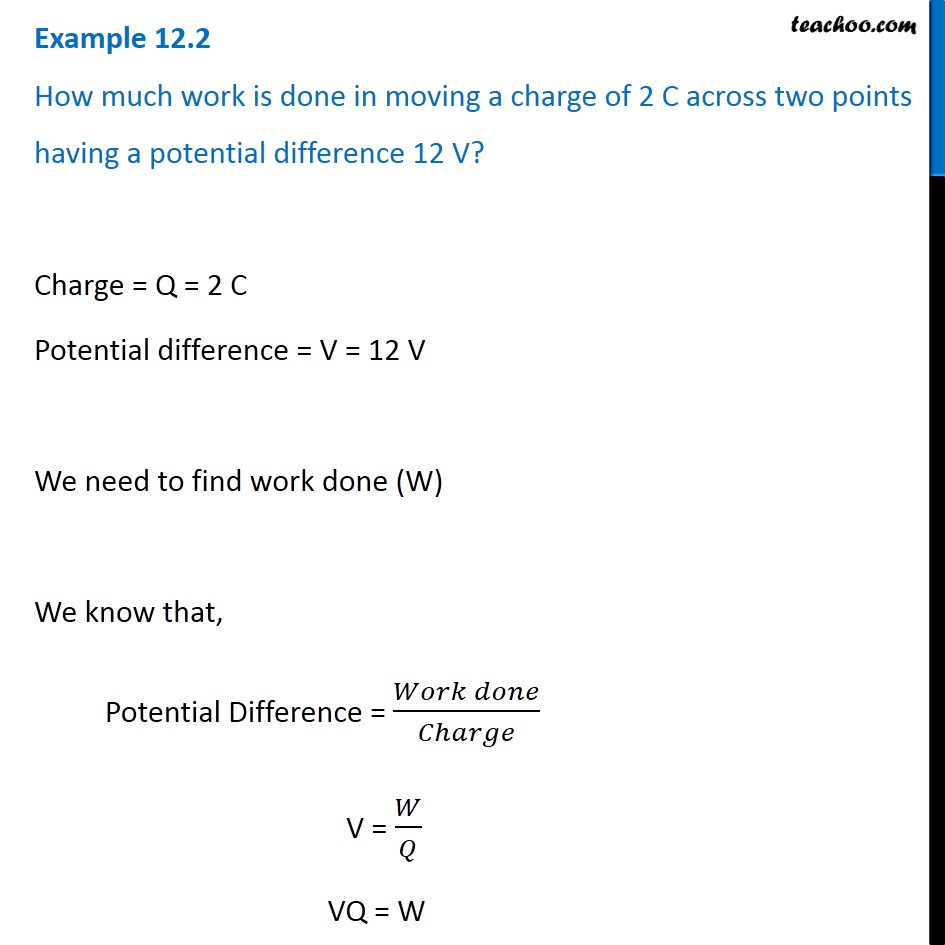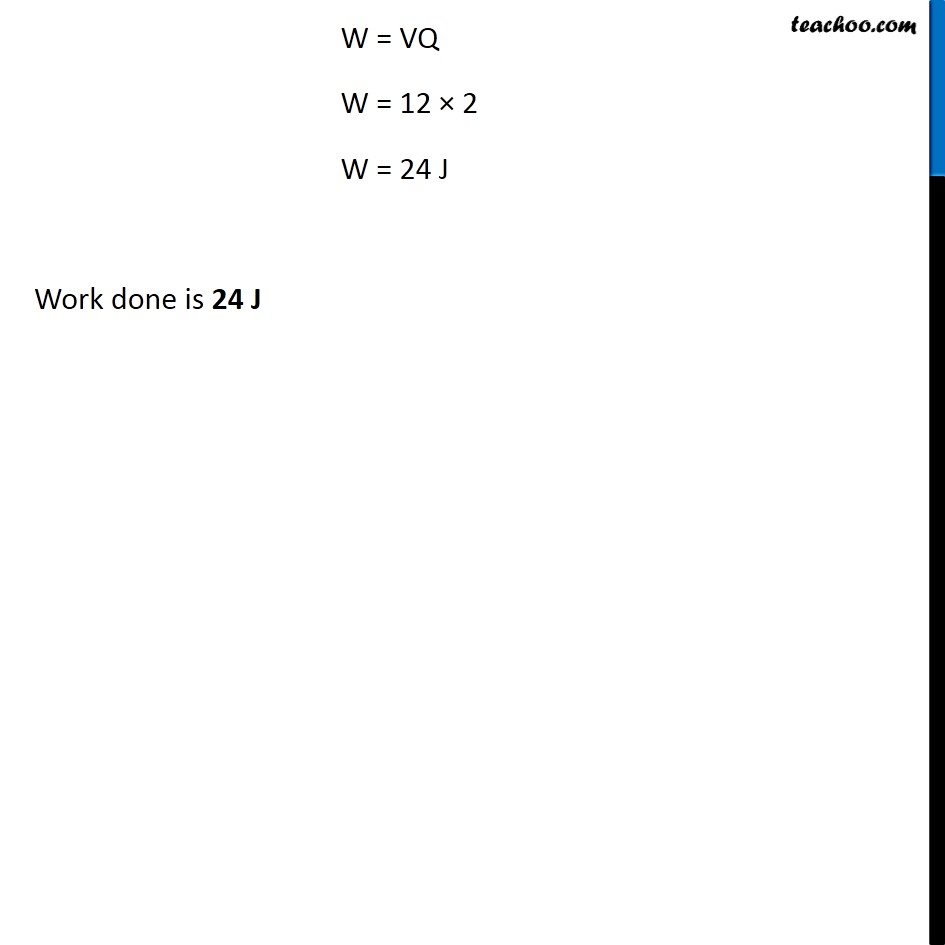Examples from NCERT Book

Class 10
Chapter 12 Class 10 - Electricity

## How much work is done in moving a charge of 2 C across two points having a potential difference 12 V?Learn in your speed, with individual attention - Teachoo Maths 1-on-1 Class

### Transcript

Example 12.2 How much work is done in moving a charge of 2 C across two points having a potential difference 12 V? Charge = Q = 2 C Potential difference = V = 12 V We need to find work done (W) We know that, Potential Difference = (𝑊𝑜𝑟𝑘 𝑑𝑜𝑛𝑒)/𝐶ℎ𝑎𝑟𝑔𝑒 V = 𝑊/𝑄 VQ = W W = VQ W = 12 × 2 W = 24 J Work done is 24 J